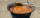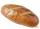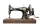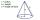# Colza

In the agricultural cooperative harvested 525 ares of colza, of which received 5.6 tons of seeds. Calculate the yield per hectare.

Result

x =  1.067 t/ha

#### Solution:Leave us a comment of example and its solution (i.e. if it is still somewhat unclear...):

Showing 0 comments:Be the first to comment!#### To solve this example are needed these knowledge from mathematics:

Do you want to convert area units? Do you want to convert mass units?

## Next similar examples:

1. RapeThe agricultural cooperative harvested 525 ares of rape, of which received 5.6 tons of rape seeds. Calculate the yield per hectare of rape.
2. Pizza 4Marcus ate half pizza on monday night. He than ate one third of the remaining pizza on Tuesday. Which of the following expressions show how much pizza marcus ate in total?
3. Street numbersLada came to aunt. On the way he noticed that the houses on the left side of the street have odd numbers on the right side and even numbers. The street where he lives aunt, there are 5 houses with an even number, which contains at least one digit number 6.
4. RecipeA recipe requires 2 pounds of flour. If a chef wants to triple the recipe, how many ounces of flour will be needed?
5. Pound2kilosHow many pounds make 1 kilograms?
6. AreaCalculate: ?
7. One hectareHow many square meters are one hectare?
8. StewTo prepare stew for 10 loggers we need: 2.5 kg of potatoes, 0.8 kg of meat, 18 dag of flour, 30 dag onion and 1 bread. What is the required amount of raw materials for 50 participants camps where loggers eats 1.5 times more than a participant of the camp?
9. Customary lengthConvert length 65yd 2 ft to ft
10. Bread5 of the same bread has the same weight as three bread and 4 kg of fruit. What weight has one bread?
11. SewingThe lady cut off one half of cloth. She needed three-quarters of this piece to sew a skirt. What part of the original piece of cloth still remained?
12. Find xSolve: if 2(x-1)=14, then x= (solve an equation with one unknown)
13. RainfallHow many liters of water did fell in a 32m long and 8m wide garden, if 8mm of rain fell?
14. The diagramThe diagram is a cone of radius 8cm and height 10cm. The diameter of the base is. ..
15. Dropped sheetsThree consecutive sheets dropped from the book. The sum of the numbers on the pages of the dropped sheets is 273. What number has the last page of the dropped sheets?
16. LandLand has a rectangular shape, its surface area is 1.45 hectares. Its width is 250 m. Determine the length of the land.
17. DisjointHow many elements have union and intersection of two disjoint sets when the first have 1 and secodn 8 elements.